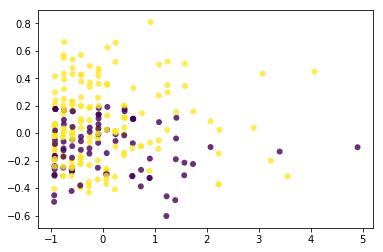# Factor Analysis in Python

Factor analysis is a dimensionality reduction technique commonly used in statistics. FA is similar to principal component analysis. The difference are highly technical but include the fact the FA does not have an orthogonal decomposition and FA assumes that there are latent variables and that are influencing the observed variables in the model. For FA the goal is the explanation of the covariance among the observed variables present.Our purpose here will be to use the BioChemist dataset from the pydataset module and perform a FA that creates two components. This dataset has data on the people completing PhDs and their mentors. We will also create a visual of our two-factor solution. Below is some initial code.

```import pandas as pd
from pydataset import data
from sklearn.decomposition import FactorAnalysis
import matplotlib.pyplot as plt```

We now need to prepare the dataset. The code is below

```df = data('bioChemists')
df=df.iloc[1:250]
X=df[['art','kid5','phd','ment']]```

In the code above, we did the following

1. The first line creates our dataframe called “df” and is made up of the dataset bioChemist
2. The second line reduces the df to 250 rows. This is done for the visual that we will make. To take the whole dataset and graph it would make a giant blob of color that would be hard to interpret.
3. The last line pulls the variables we want to use for our analysis. The meaning of these variables can be found by typing data(“bioChemists”,show_doc=True)

In the code below we need to set the number of factors we want and then run the model.

```fact_2c=FactorAnalysis(n_components=2)
X_factor=fact_2c.fit_transform(X)```

The first line tells Python how many factors we want. The second line takes this information along with or revised dataset X to create the actual factors that we want. We can now make our visualization

To make the visualization requires several steps. We want to identify how well the two components separate students who are married from students who are not married. First, we need to make a dictionary that can be used to convert the single or married status to a number. Below is the code.

```thisdict = {
"Single": "1",
"Married": "2",}```

Now we are ready to make our plot. The code is below. Pay close attention to the ‘c’ argument as it uses our dictionary.

`plt.scatter(X_factor[:,0],X_factor[:,1],c=df.mar.map(thisdict),alpha=.8,edgecolors='none')`You can perhaps tell why we created the dictionary now. By mapping the dictionary to the mar variable it automatically changed every single and married entry in the df dataset to a 1 or 2. The c argument needs a number in order to set a color and this is what the dictionary was able to supply it with.

You can see that two factors do not do a good job of separating the people by their marital status. Additional factors may be useful but after two factors it becomes impossible to visualize them.

Conclusion

This post provided an example of factor analysis in Python. Here the focus was primarily on visualization but there are so many other ways in which factor analysis can be deployed.

## 1 thought on “Factor Analysis in Python”

1.Wakhinuddin Wakhid

Thank you